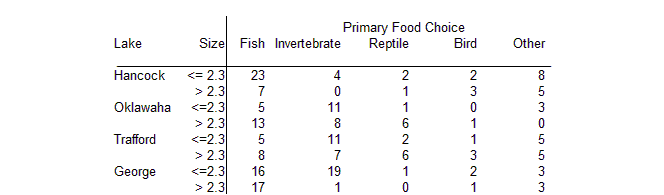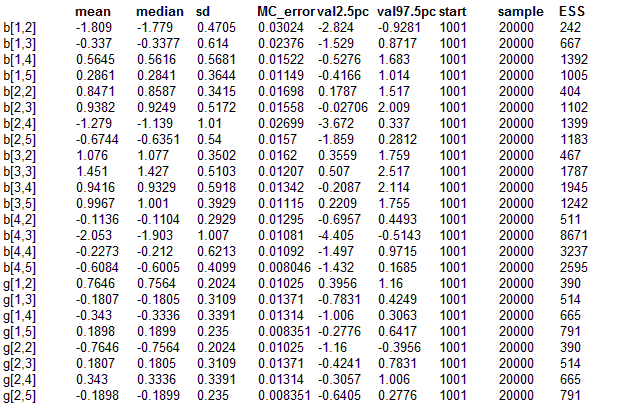#### Alligators: multinomial - logistic regression

Agresti (1990) analyses a set of data on the feeding choice of 221 alligators, where the response measure for each alligator is one of 5 categories: fish, invertebrate, reptile, bird, other. Possible explanatory factors are the length of alligator (two categories: <= 2.3 metres and > 2.3 metres), and the lake (4 catgeories: Hancock, Oklawaha, Trafford, George). The full data is shown below.Each combination of explanatory factors is assumed to give rise to a multinomial response with a logistic link, so that for lake i, size j, the observed vector of counts Xij. = Xij1,...,Xij5 has distribution

Xij. ~ Multinomial(pij.,nij)
pijk = φijk / Σk φijk
φijk = eαk + βik + γjk

where nij = Σk Xijk, and α1, βi1, β1k, γj1, γ1k = 0 for identifiability. This model is discussed in detail in the Classic BUGS manual (version 0.5) in the section on Multionomial LogisticModels. All unknown α's, β's , γ's are initially given independent "noninformative" priors.

The Classic BUGS manual (version 0.5) discusses two ways of fitting this model: directly in the form given above or by using the multinomial-Poisson transformation which will be somewhat more efficient. Both techniques are illustrated in the code given below.

model
{

# PRIORS
alpha <- 0; # zero contrast for baseline food
for (k in 2 : K) {
alpha[k] ~ dnorm(0, 0.00001) # vague priors
}
# Loop around lakes:
for (k in 1 : K){
beta[1, k] <- 0
} # corner-point contrast with first lake
for (i in 2 : I) {
beta[i, 1] <- 0 ; # zero contrast for baseline food
for (k in 2 : K){
beta[i, k] ~ dnorm(0, 0.00001) # vague priors
}
}
# Loop around sizes:
for (k in 1 : K){
gamma[1, k] <- 0 # corner-point contrast with first size
}
for (j in 2 : J) {
gamma[j, 1] <- 0 ; # zero contrast for baseline food
for ( k in 2 : K){
gamma[j, k] ~ dnorm(0, 0.00001) # vague priors
}
}

# LIKELIHOOD
for (i in 1 : I) { # loop around lakes
for (j in 1 : J) { # loop around sizes

# Multinomial response
# X[i,j,1 : K] ~ dmulti( p[i, j, 1 : K] , n[i, j] )
# n[i, j] <- sum(X[i, j, ])
# for (k in 1 : K) { # loop around foods
# p[i, j, k] <- phi[i, j, k] / sum(phi[i, j, ])
# log(phi[i ,j, k]) <- alpha[k] + beta[i, k] + gamma[j, k]
# }

# Fit standard Poisson regressions relative to baseline
lambda[i, j] ~ dflat()   # vague priors
for (k in 1 : K) { # loop around foods
X[i, j, k] ~ dpois(mu[i, j, k])
log(mu[i, j, k]) <- lambda[i, j] + alpha[k] + beta[i, k] + gamma[j, k]
cumulative.X[i, j, k] <- cdf.pois(X[i, j, k], mu[i, j, k])
}
}
}

# TRANSFORM OUTPUT TO ENABLE COMPARISON
# WITH AGRESTI'S RESULTS

for (k in 1 : K) { # loop around foods
for (i in 1 : I) { # loop around lakes
b[i, k] <- beta[i, k] - mean(beta[, k]); # sum to zero constraint
}
for (j in 1 : J) { # loop around sizes
g[j, k] <- gamma[j, k] - mean(gamma[, k]); # sum to zero constraint
}
}
}

##### Data
``` list( I = 4, J = 2, K = 5,   X = structure(.Data = c(23, 4, 2, 2, 8, 7, 0, 1, 3, 5, 5, 11, 1, 0, 3, 13, 8, 6, 1, 0,                        5, 11, 2, 1, 5, 8, 7, 6, 3, 5, 16, 19, 1, 2, 3, 17, 1, 0, 1, 3), .Dim = c(4, 2, 5))) ```
##### Inits for chain 1
``` list(alpha = c(NA, 0, 0, 0, 0),   beta = structure(.Data = c(NA, NA, NA, NA, NA,                        NA, 0, 0, 0, 0,                        NA, 0, 0, 0, 0,                        NA, 0, 0, 0, 0), .Dim = c(4, 5)),      gamma = structure(.Data = c(NA, NA, NA, NA, NA,                              NA, 0, 0, 0, 0), .Dim = c(2, 5)),      lambda = structure(.Data = c(0, 0, 0, 0,                           0, 0, 0, 0), .Dim = c(4, 2))) ```

##### Inits for chain 2
``` list(alpha = c(NA, 1, 1, 1, 1),   beta = structure(.Data = c(NA, NA, NA, NA, NA,                        NA, 2, 2, 2, 2,                        NA, 2, 2, 2, 2,                        NA, 2, 2, 2, 2), .Dim = c(4, 5)),      gamma = structure(.Data = c(NA, NA, NA, NA, NA,                              NA, 3, 3, 3, 3), .Dim = c(2, 5)),      lambda = structure(.Data = c(4, 4, 4, 4,                           4, 4, 4, 4), .Dim = c(4, 2))) ```
Results